Get instant live expert help with Excel or Google Sheets“My Excelchat expert helped me in less than 20 minutes, saving me what would have been 5 hours of work!”

#### Post your problem and you’ll get Expert help in seconds.

Your message must be at least 40 characters
Our professional Expert are available now. Your privacy is guaranteed.

# How to use the Excel WHAT IF analysis

The What if analysis tool  is a useful method for testing various outcomes in Excel.

We can examine things in different scenarios by using whatif Excel functionality.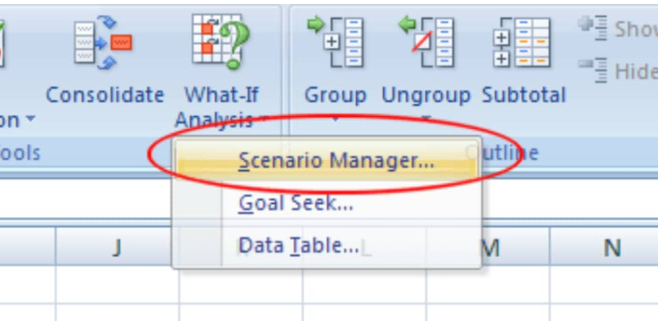Figure 1. What If Analysis in Excel

By making use of Excel What-If Analysis tools, we can include various sets of data in our formula(s) to explore every possible result.

## Definition of What If Analysis

Excel what-if analysis is one of many features we can use to perform advanced mathematical calculations. The Excel what if feature can help us test and answer questions using specified data sets, even when the available information is incomplete.

## How to do a What If Analysis in Excel

When we generate an Excel function or formula, we put various components together to return a desired result. The Goal Seek function in Excel operates in an opposite manner: It allows us to begin with our desired result, and then it determines the input value which will give us our desired result. We will now demonstrate how we can use Goal Seek to carry out What if analysis in Excel.

Let’s assume that we have enrolled for a class. We currently have a 65 score, but we require a minimum score of 70 to pass our class. Luckily, we have one last assignment that may be able to increase our average. We can use the Goal Seek feature to discover exactly what score we need on our last assignment to pass our class.

1. In our worksheet example below, we have collected the data available to us from our first four assignments (see column A). Even though we do not know the outcome of our fifth test, we can enter a function or formula that will calculate our final grade. The average formula we will enter into cell B7 of our worksheet example is as follows;
`=AVERAGE(B2:B6)`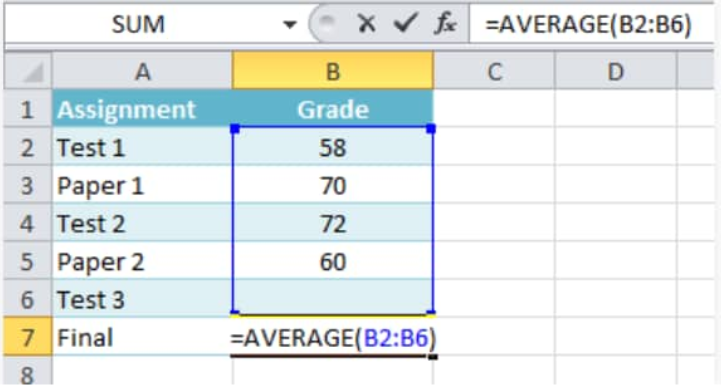Figure 2. Average Function in Excel

Our purpose here is to use the Goal Seek feature,  to determine the minimum grade we will require on our final assignment and display it in in cell B6 of our worksheet example.

1. Next we will select the cell which contains the value we desire to change.
When we use Goal Seek, we will have to choose a cell which already contains a function or formula.
In our worksheet example, we will select cell B7 since it already contains a formula;
`=AVERAGE(B2:B6)`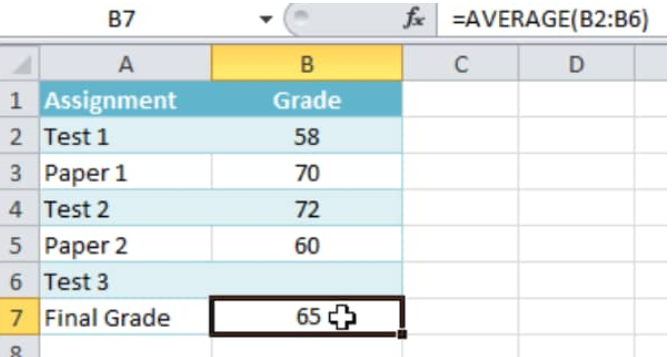Figure 3. Cell Selection for Goal Seek in Excel

1. Click on the “Data” tab, and then click on the “What-If Analysis” button, and then select the “Goal Seek” option from the fly-out menu: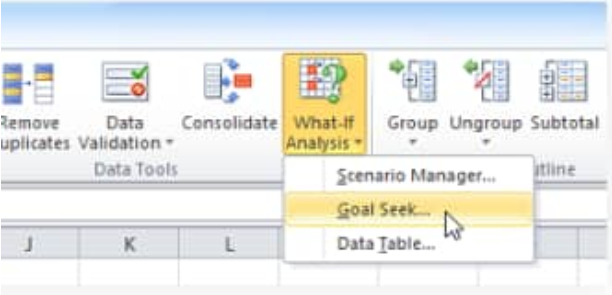Figure 4. Goal Seek Button in Excel

A dialog box should appear with 3 fields:

• Set cell = The cell which will contain our desired outcome. In our worksheet example, cell B7 has already been selected.
• To value = This is our desired outcome. In our worksheet example, we will enter 70 since we must earn that minimum score to pass our class.
• By changing cell = The cell where the Goal Seek feature will return our answer. In our worksheet example, we will choose cell B6 since we want to calculate the grade we must score on our final assignment.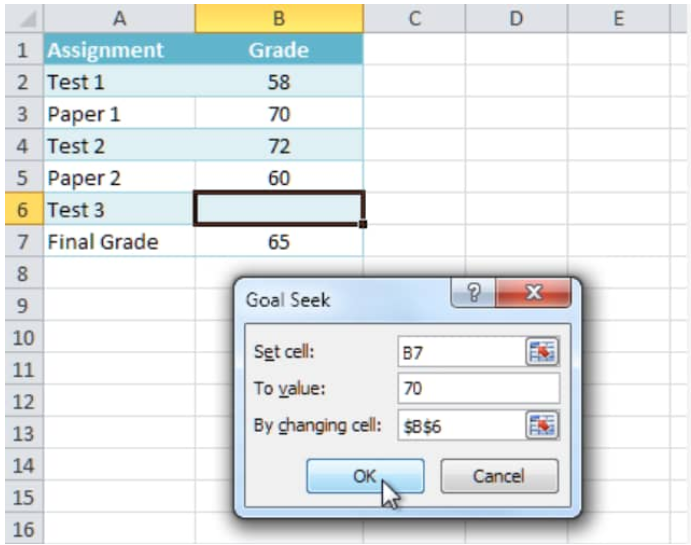Figure 5. Goal Seek Menu

1. We can click “OK” when we are done: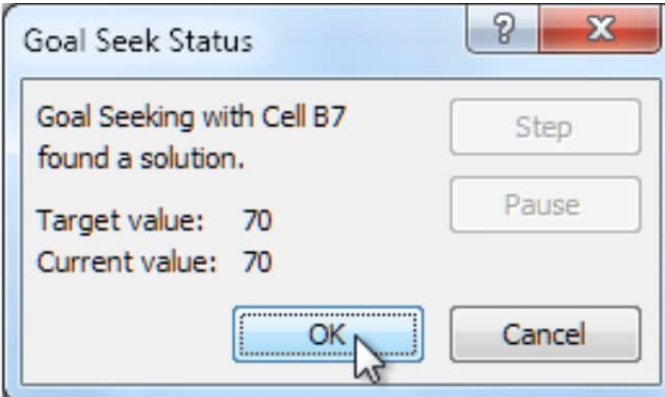Figure 6. of Goal Seek Outcome

1. Our predicted result will appear inside the cell we have selected. In our worksheet example, Goal Seek determined that we must score a minimum of 90 in our final test to earn a pass;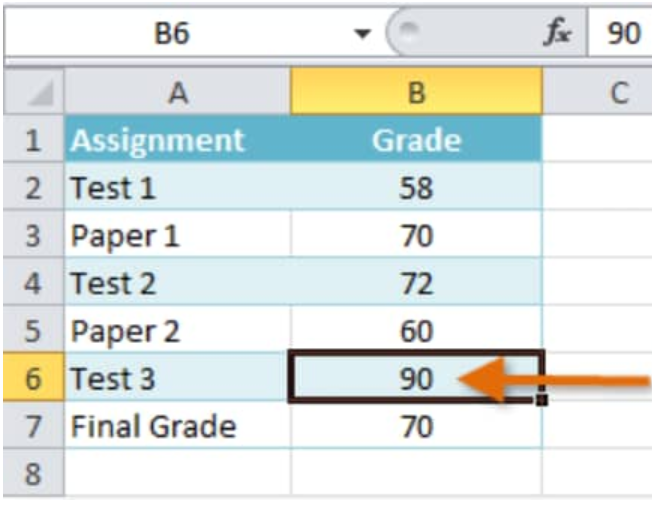Figure 7. What If Analysis in Excel

## Instant Connection to an Excel Expert

Most of the time, the problem you will need to solve will be more complex than a simple application of a formula or function. If you want to save hours of research and frustration, try our live Excelchat service! Our Excel Experts are available 24/7 to answer any Excel question you may have. We guarantee a connection within 30 seconds and a customized solution within 20 minutes.